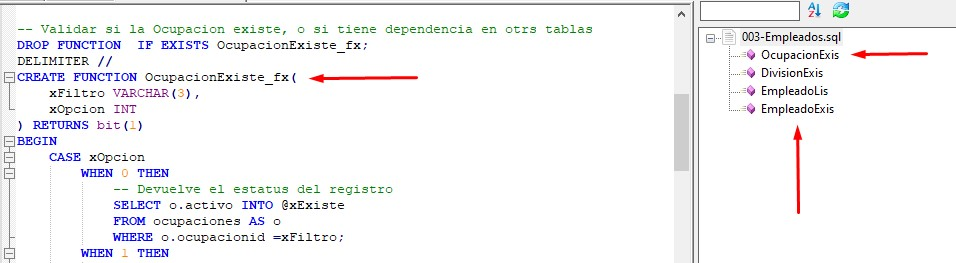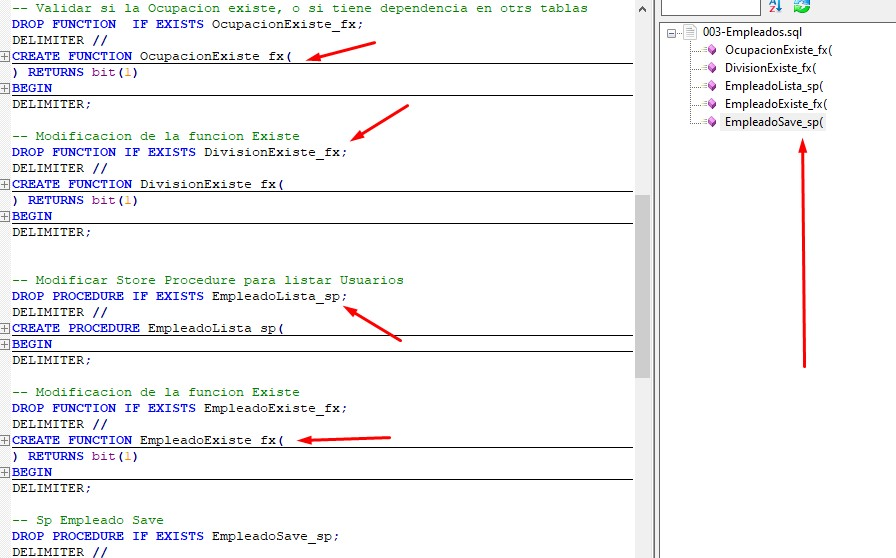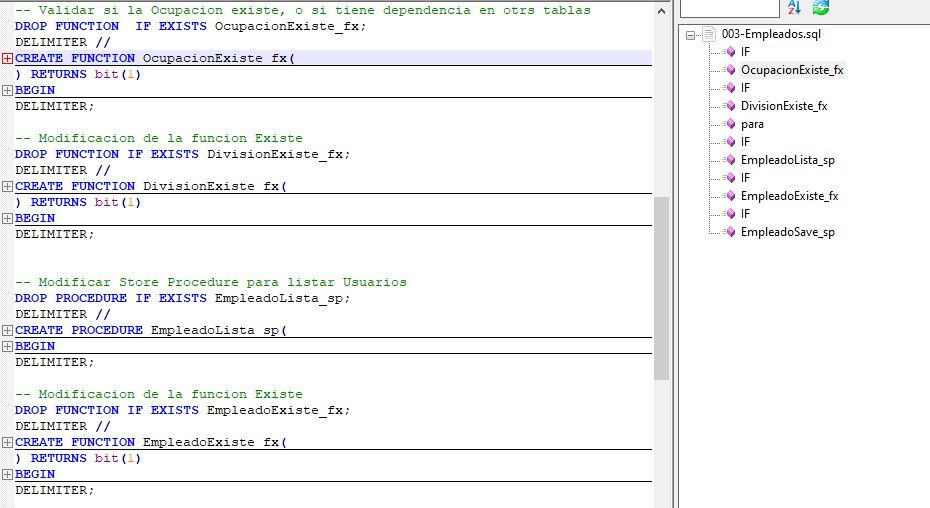• Hi everyone, I’m lloking for some hel abut this topic, I developed a VBA application using MariaDB database, now I’m updating the database structure because i change some vba code, I’m using notepad++ to write de sql scrip, and Iwould like to have the list functions of my scrip, but the sql function list of notepad++ only showme a part of my function and Sp.
Someome could help me, what I have to do?, I don’t know nothing about regular expression . Thans for your help.• I see the same thing.

It looks like the `([\s]*([ia]s))` alternative is hitting, and matching on i or a followed by s, which hits at the “is” in each of those function names. I don’t know why an SQL function-name parser would want that.

I changed those to be `\w+` instead of `[ia]s` , and at least for my example, it worked.

But since I don’t SQL very well, I don’t know if that would break anything.

To implement this:

2. Edit `%AppData%\Notepad++\functionList\sql.xml`, and make it look like:
``````<NotepadPlus>
<functionList>
<!-- ========================================================= [ PL/SQL ] -->
<parser id="plsql_function" displayName="PL/SQL" commentExpr="((/\*.*?\*)/|(//.*?\$))">
<function
mainExpr="((PROCEDURE)|(FUNCTION))[\s]+[A-Za-z][\w_]*([\s]*\([^\)]*\)[\s]*)?(([\s]*;)|([\s]*(\w+))|([\s]+(RETURN)([\s]+[\w%\.]+)+(([\s]*;)|([\s]+(\w+)))))"
displayMode="\$className->\$functionName"
>
<functionName>
<nameExpr expr="[\s]+[A-Za-z][\w_]*([\s]*\([^\)]*\))?(([\s]*;)|([\s]*(\w+))|([\s]+(RETURN)([\s]+[\w%\.]+)+(([\s]*;)|([\s]+(\w+)))))"/>
<nameExpr expr="[A-Za-z][\w_]*([\s]*\([^\)]*\))?(([\s]*;)|([\s]*(\w+))|([\s]+(RETURN)([\s]+[\w%\.]+)+(([\s]*;)|([\s]+(\w+)))))"/>
<nameExpr expr="[A-Za-z][\w_]*([\s]*\([^\)]*\))?(([\s]+(RETURN)([\s]+[\w%\.]+)+))*"/>
</functionName>
</function>
</parser>
</functionList>
``````
1. Save and exit

(Personally, I think the expressions they use could use some cleanup; a lot of parens that aren’t doing anything, and made it hard for me to figure out even as much as I did. )

You also might want to look at @MAPJe71’s repo which includes functionList XML files for bunches of languages – if there’s an SQL in that repo, it might work better than yours. https://github.com/MAPJe71/Languages (I am seeing a parameter-completion file, but not any functionList file, unfortunately).

• @PeterJones I was changing those code, but I did on C:\programs files, I changed the code using www.regex.com, and the code works, I leave the code and the pict as how I wanted. Thanks so much, and Excuseme by my english skill…,```
<functionList>
<!-- ===== [ PL/SQL ] -->
<parser id=“plsql_function” displayName=“PL/SQL” commentExpr="((/*.?*)/|(//.?\$))">
<function
mainExpr="((CREATE PROCEDURE)|(CREATE FUNCTION))[\s]+[A-Za-z][\w_][\wfx]([\s]([^)]|([\s]+(RETURNS)))"
displayMode="\$className->\$functionName"
>
<functionName>
<nameExpr expr="[\s]+[A-Za-z][\w_][\wfx]([\s]([^)]|([\s]+(RETURNS)))"/>
</functionName>
</function>
</parser>
</functionList>

• I know you found one that worked for you. But I found another couple places:

• @PeterJones Did you use @MAPJe71 code?, I going to test rigth now, it’s the result, its show the IF sentenses and some code where be in comment.
I going to use the code that I changed, because I need only Functions and Store procedure, and I ever use the _fx() for Functions and _sp for Store Procedure.
sincerely. I’m very greatifull for your help.

• I forget to add how the image looks